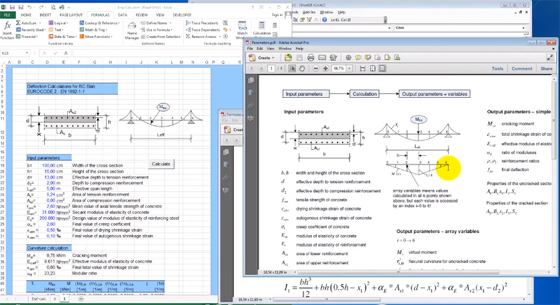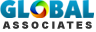# How to apply excel for performing various engineering calculationThe engineers require to perform several calculations on daily basis specifically with modern standards like EUROCODES. Microsoft excel with its powerful programming features can make this engineering calculation process simple.

Microsoft excel contains various input parameters which can be entered in very easy to follow and rapid manner. Besides, with excel, a simple sketch can be provided as a graphical representation concerning the basis of the computation with annotations of input parameters. Excel program also facilitates the Engineers to describe the mathematical process with some complicated formulas or iterations.

In this regard, GrSoft Structural Engineering is conducting a useful course for engineers to produce their own customized engineering calculations instantly as well as update formulas in their calculation whenever needed through robust programmable tools in excel.

The course will focus on the following subjects :-

- How to develop cells where input parameters should be entered
- How to develop a sketch involving anotations of input parameters
- How to arrange cells where calculation results will be written
- How to make a push button, where you will activate start of the

OTHER FEATURES:

calculation:

- How to write code for doing calculation
- How to write code to demonstrate the calculation results
- How to execute calculationImage Courtesy: cesdb.co

This course will also provide guidance to write the software for practical engineering computation for structural analysis. One will also be familiar with how to enter data, describe formulas and make calculation real time along with how to demonstrate results and format cells for results of calculation.

# Estimating Sheets